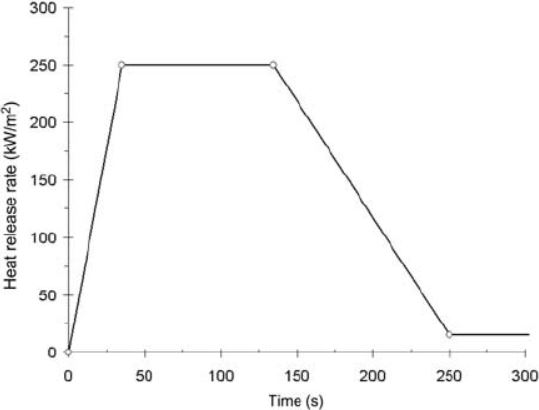## Fluids

•hgsdvd
Subscriber

Hello Guys,

I am facing an obstacle now in writing an expression to define a chart as a function of Height. I have written the expression and has gone smoothly except that the problem with the units i guess. I keep receiving a message telling me that there is an inconsistent dimensions in the expression.

Could anybody figure out the problem?

I really appreciate any help.

Thanks

Expressions:

Fire Height= 1[m] *((3.7[m] *(FireProfile^(2/5)))-1.02[m])

Fire Profile= if(t<3300, if(t<600, FireGrowth, FirePower), FireExtinction)

The message

Inconsistent dimensions on each side of '-' operator at position 37.

Dimensions on left: 'kg^0.400 m^1.800 s^-1.200'

Dimensions on right: 'm'.

•raul.raghav
Subscriber
Muhannad, the units for your expression of “Fire Profile” is [kg m^2 s^-3]. You can’t subtract these units with [m] in your “Fire Height” expression. Based on the way you have defined your “Fire Height” expression, the expression “Fire Profile” needs to be dimensionless. If “Fire Profile” is dimensionless, your “Fire Height” would have units of [m^2]. Make sure you check all this.
•hgsdvd
Subscriber

Dear Rahul,

Thanks for you reply. I am trying to define the "Fire Profile" expression according to the attached graph. As far as I know, I have to insert some units to let the solver reads it correctly, unless if there is another way to do so, then I will appreciate knowing it. Just to make it clear, the message I am receiving is referring to the expressions below, which the expressions above are a part of. However, the reason why I post the " Fire Height" expression is that I started facing this warnings once I defined the "Fire Height" expression.

Expressions:

heatSource = insideSource*FireProfile/max(SourceVolume, minVolume)

Thank you•raul.raghav
Subscriber

Muha, its still not clear how you're trying to define the expressions. The issue was regarding the "Fire Height" variable you defined.

Fire Height= 1[m] *((3.7[m] *(FireProfile^(2/5)))-1.02[m])

[*error*] = [m] * ( ([kg^0.4 m^1.8 s^-1.2]) - [m] )

The error is because you're trying to subtract [kg^0.4 m^1.8 s^-1.2] and [m]. You need to be dimensionally consistent.

•hgsdvd
Subscriber

I got your point. So, any suggestion on how it be possible to use the "Fire Height" equation as a function of the "Fire Profile" expression whether by CEL expressions or different ways?

Thanks

Muha,

•raul.raghav
Subscriber

Muha, you have to provide more information on what you're trying to achieve. A reference would be of help to understand what you're trying to do.

•DrAmine
Ansys Employee

Please tell us what you want to do and what you have as input.

•raul.raghav
Subscriber

Muha, your equation for "Fire Profile" should be composed of Eqn's (1), (2) and (3), which I believe you did correctly. The equation you define for "Fire Height" requires the non-dimensional form of heat release rate (HRR) and you have to find information regarding how your "Fire Profile" equation is being non-dimensionalized.

And the equation "Fire Profile" can be defined as follows:

Fire Profile = if(t<600, FireGrowth, if(t<3300, FirePower, FireExtinction))